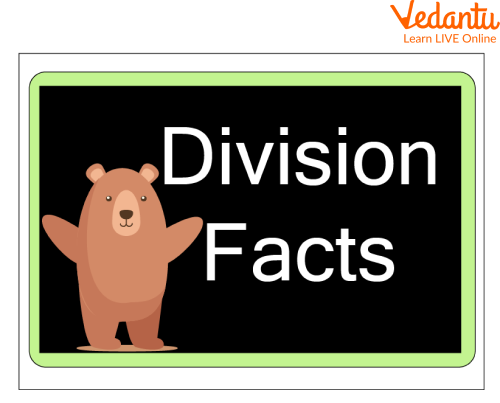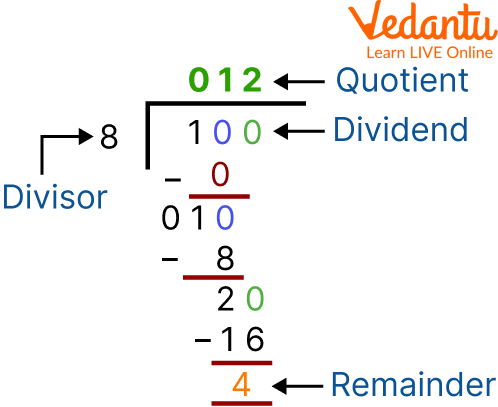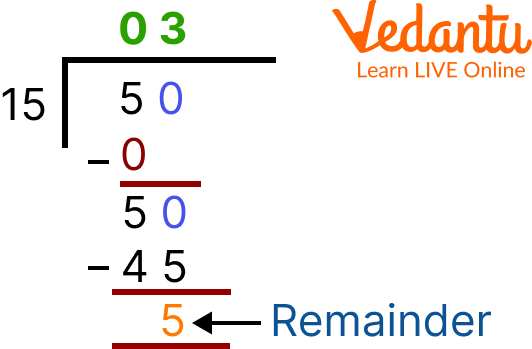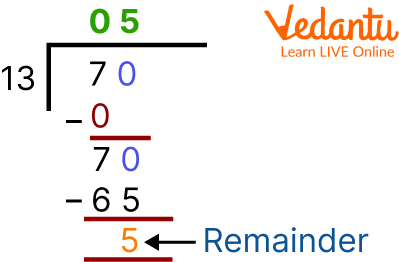Courses
Courses for Kids
Free study material
Free LIVE classes
More

# Division Facts Worksheets## Introduction to Long Division Worksheets

Last updated date: 24th Mar 2023
Total views: 26.7k
Views today: 0.09k

You must have heard your mathematics teacher talk about division. But do you know what division facts are? As we all know, the division is one of the four basic pillars of math. In very simple words, division can be defined as a process that helps in sharing a collection of items or numbers into equal parts and finding out how many equal parts can be made. In this article, we will learn what division facts are with the help of division facts worksheets. Here we will also solve the long division practice worksheet to get hold of the concepts.Division Facts

## Division Facts

Multiplication tables are one of the most important concepts as far as basic mathematics is concerned. Right from the first grade and above, children have multiplication tables to learn from their mathematics textbooks. If one knows multiplication tables, it will be easier to understand the division facts.

Division facts are division number sentences related to the knowledge of multiplication tables.

For example, 40 4 = 10, 20 4 = 5, and 8 4 = 2 are all division facts of the four. Let us understand more about the division facts with the help of a long division practice worksheet.

## Long Division (Worksheet)

Mathematically, long division is a method of dividing large numbers into smaller parts or groups. It helps to break a problem into small and simple steps. Dividends, divisors, quotients, and remainders are important parts of long division. In a problem relating to long division, the dividend is the large number divided by another number called the divisor. The quotient is the result of the division, and the excess quantity or number which cannot be divided is known as the remainder.An Example From Math Division Worksheets

## 2-Digit Division

The division is a tool that is used to distribute objects or numbers among different people in equal parts. Let's go through the division of 2-digit numbers in detail, along with 2-digit division worksheets. Here is the process of 2-digit division in a step-by-step manner:

• Let’s take the first digit of the dividend, which should be equal to the number of digits the divisor carries.

• The number borrowed from the dividend is smaller than the divisor, and then we take the next digit of the dividend.

• The result of that division has to be written in the space of quotients.

• The digit of the quotient is multiplied by the divisor, and the result is written below the dividend and subtracted.

• In case the dividend is a smaller number, we cannot do so. Therefore, we need to choose a smaller number in the quotient until we can subtract it.

• Once we perform the subtraction, use the next digit of the dividend and repeat the division process until there are no more remaining numbers present in the dividend.

## Solved Examples

Q 1. Divide 50 by 15.

Ans. The number 15 is multiplied by the number 3 so that it is equal to 45.

50 - 45 = 5

Quotient = 3

Remainder = 5

Thus, the quotient and the remainder of the division of 50 and 15 are 3 and 5, respectively.Divide 50 by 15

Q 1. Divide 70 by 13.

Ans. The number 13 is multiplied by the number 5 to equal to 65.

70 - 65 = 5

Quotient = 5

Remainder = 5

Thus, the quotient and the remainder of the division of 70 and 13 are 5 and 5, respectively.2-digit Division

Q 1. 56 8 =?

Ans: 7

Q 2. 44 4 =?

Ans: 11

Q 3. 70 7 =?

Ans: 10

## 2-Digit Division WorksheetsLong Division Practice Worksheet

## Long Division WorksheetsLong Division Practice Worksheet

## Summary

In this article, we learned and discussed division facts along with long division worksheets, 2-digit division worksheets, and other math division worksheets. We have also referred to some solved examples to understand the concept of the topics in a very convenient manner. Along with that, we tried to explain what division facts are and how they are practised. We have learned to solve long divisions and also the 2-digit divisions. We hope now the children have understood all the concepts and they enjoy solving the math division worksheets even more.

## FAQs on Division Facts Worksheets

1. Can the divisor be Zero?

No, the divisor can not be 0 because, in mathematics, division by the number 0 is not defined.

2. How can the quotient and the remainder of the division be verified?

The quotient and the remainder can be verified by using the division expression, which is given by (Divisor x Quotient) + Remainder = Dividend.

3. What is the difference between long division and short division?

The short division is great for dividing larger numbers by one-digit numbers, whereas the long division is used for dividing large numbers with two or more digits.